College Physics : Tension

Example Questions

Example Question #11 : Forces

Suppose that an object of a certain mass that is hanging from a wall is suspended by two ropes. Which of the following gives an expression for the tension in each rope?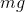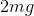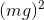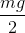Explanation:

In this question, we're told that an object is hanging by being supported by two ropes. We're asked to find the tension in each rope.

When answering this question, we have to keep in mind all of the forces acting on the object. Since the object isn't moving, there is no net force acting on it. Moreover, we know that its weight acts downward while the tension in the ropes act upwards.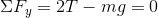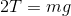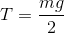Example Question #1 : Tension

An object weighing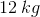is hanging from a string with no mass and a length of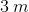. If this object is released from some initial height and reaches a maximum velocity of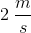, what is the maximum amount of tension in the string at any point during the object's journey?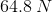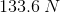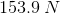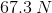Explanation:

For this question, we're told that an object hanging from a string is released from an initial height and allowed to swing. We're given the maximum velocity, the object's mass, and the length of the string. We're asked to find the maximum tension in the string.

The first step towards solving this problem is to realize that we're dealing with centripetal motion. When the object is released from an initial height, it begins to fall. But as it falls, the string exerts a tension on the mass and causes the mass to travel in a circular motion. Thus, the tension of the string is supplying the centripetal force.

Furthermore, it's important to remember the point at which the object will have maximum velocity. When the object was initially released from some initial height, all of the object's energy was in the form of gravitational potential energy. As it falls, that potential energy is converted into kinetic energy. Once the object reaches the very bottom of its path of motion, all of the potential energy it once had has been converted into kinetic energy at that instant. Since all of the energy is in the form of kinetic energy, and the object's mass remains constant, we know that this must be the point where the maximum velocity occurs.

Thus, we need to analyze the situation when the mass has swung to the very bottom of the path. At this point, the tension is providing an upward force. Moreover, the only downward force acting on the object is its weight due to gravity. The net force resulting from these two will result in the overall centripetal force on the object. We can put this into an equation as follows.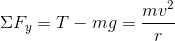Since we're trying to solve for the tension in the string, we can isolate the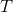term in the above expression.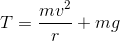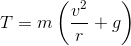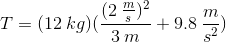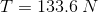All College Physics Resources